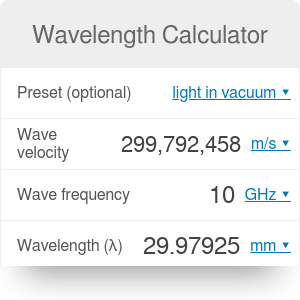Preset (optional)
light in vacuum
Wave velocity
m/s
Wave frequency
Hz
Wavelength (λ)
m

# Wavelength Calculator

By Bogna Haponiuk

The wavelength calculator can assist you in determining the relationship between frequency and wavelength. Continue reading if you are here to learn how to calculate frequency of a wave or look for the wavelength formula.

## Main properties of waves

There are three main properties of a wave: its velocity, wavelength and frequency.

Wave velocity (v) is the measure of how fast does a wave propagate in a given medium. Its unit is meter per second. Check the speed calculator for more informations about speed and velocity.

Wavelength (λ) is the distance over which the shape of wave repeats. It depends on the medium a wave travels through. It is measured in meters.

Frequency (f) of a wave refers to how many times (per a given time period) do the particles of a medium vibrate when the wave is passing through it. The unit of frequency is Hertz, or 1/second.

## Wavelength formula

The relationship between wavelength and frequency is described by this simple equation:

`λ = v/f`

Remember about proper units! If in trouble, you can always use the speed conversion.

## How to calculate wavelength

It's easy! Just use our wavelength calculator in the following way:

1. Determine the frequency of the wave. For example, `f = 10 MHz`. This frequency belongs to the radio waves spectrum.
2. Choose the velocity of the wave. As a default, our calculator uses a value of `299 792 458 m/s` - the speed of light propagating in the air.
3. Substitute these values into the wavelength equation `λ = v/f`.
4. Calculate the result - in this example, wavelength will be equal to `29.98 m`.
5. You can also use this tool as a frequency calculator. Simply type in the values of velocity and wavelength to obtain the result.

## Typical wave speeds

• Light in air or vacuum: `299 792 458 m/s`
• Light in water: `224 901 000 m/s`
• Sound in air: `343.2 m/s`
• Sound in water (20°C): `1481 m/s`
Bogna Haponiuk

## Get the widget!

Wavelength Calculator can be embedded on your website to enrich the content you wrote and make it easier for your visitors to understand your message.

It is free, awesome and will keep people coming back!